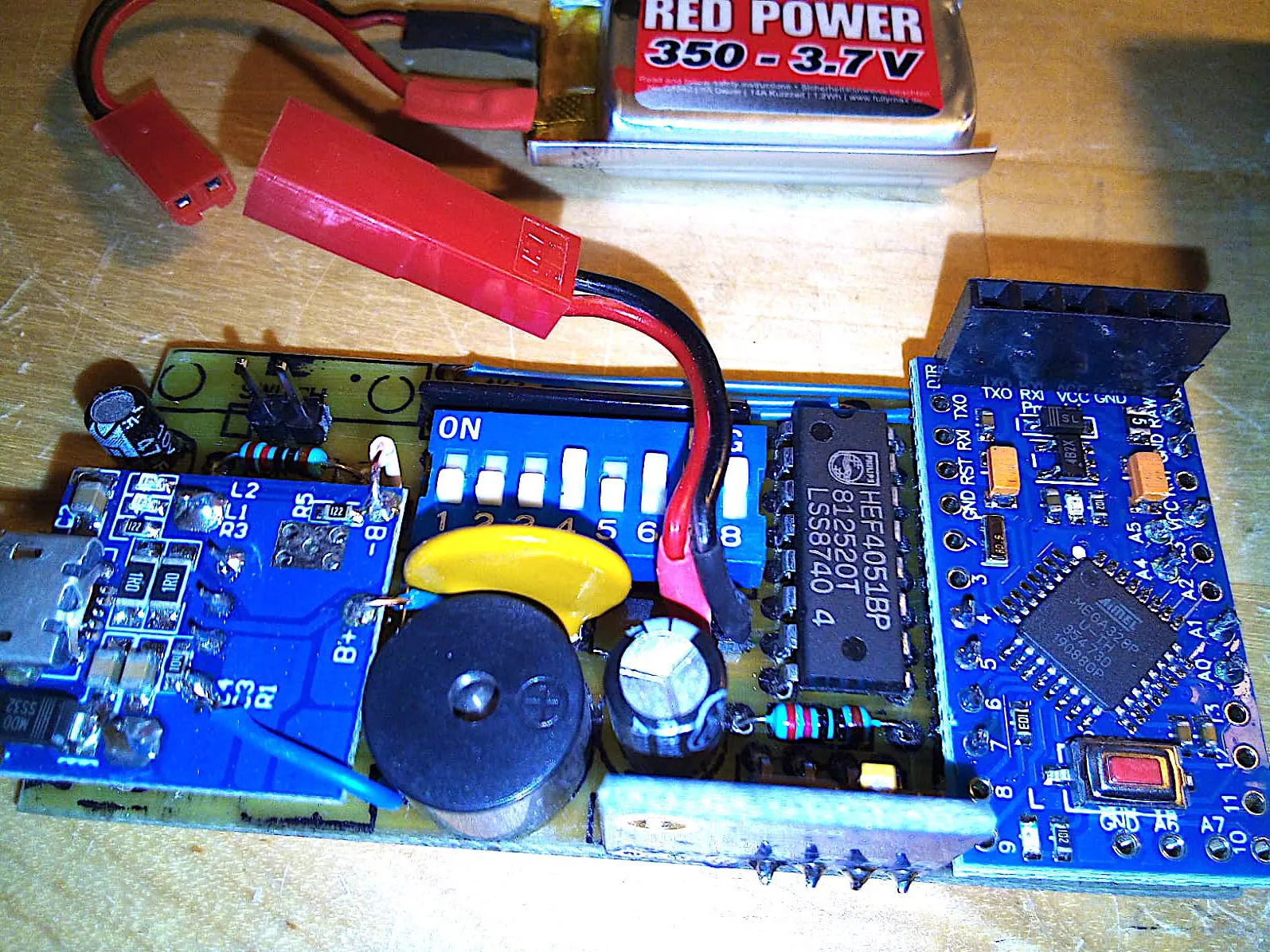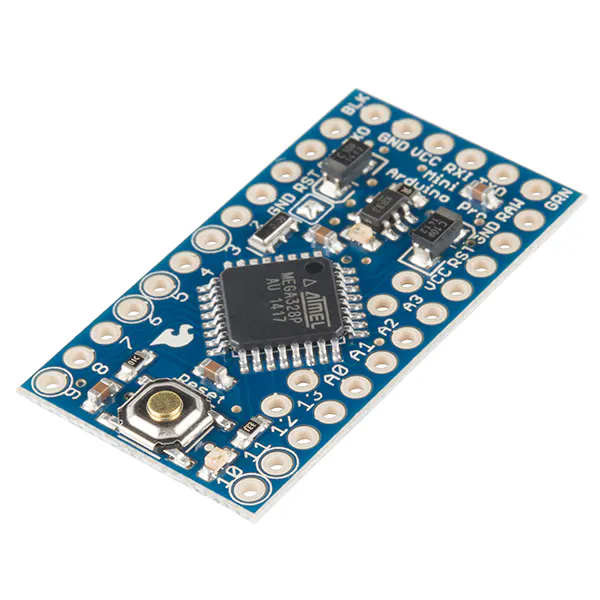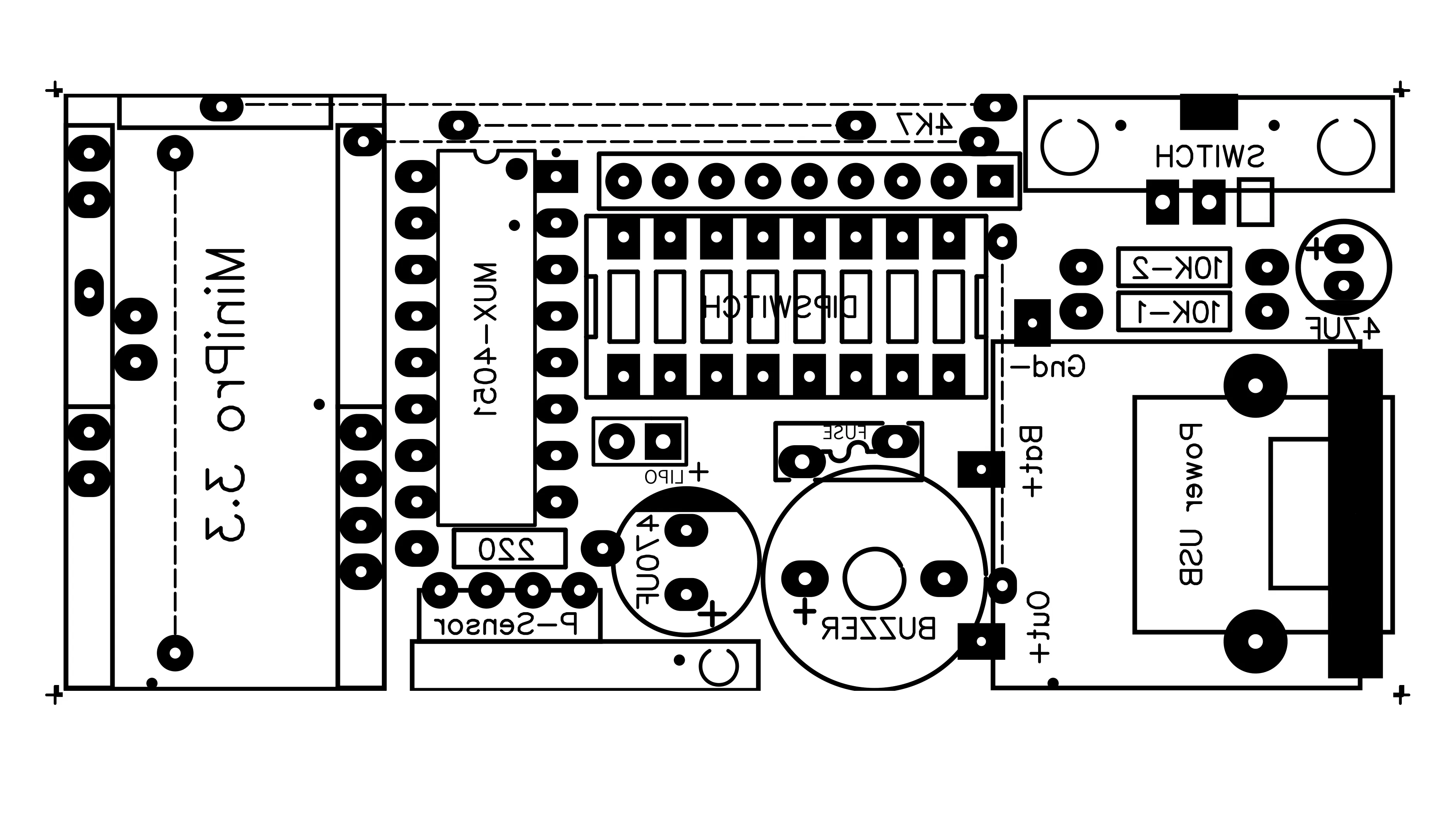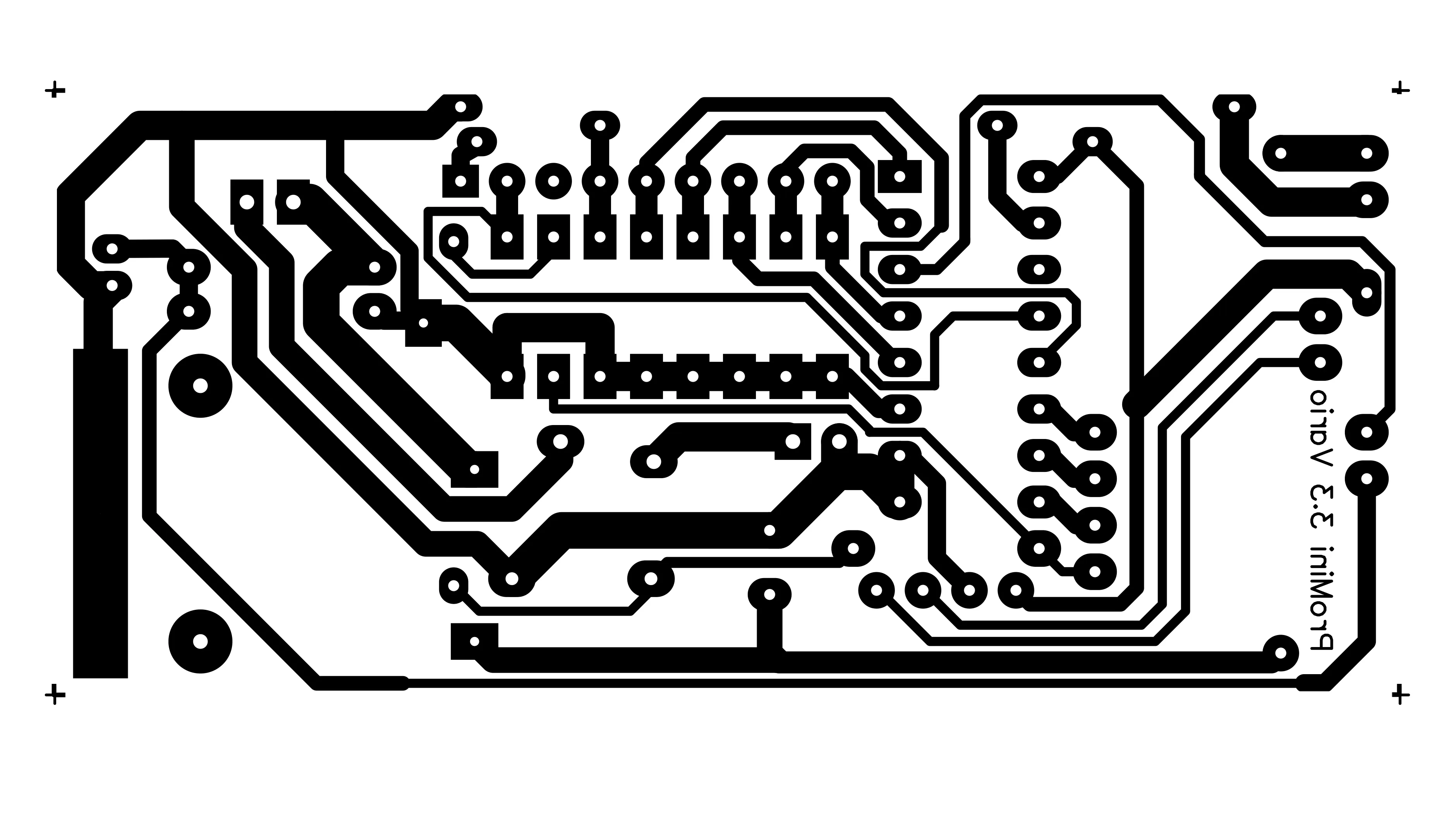# Acoustic Variometer (Ultra Sensitive Barometer)

As a free flying paragliding pilot, I have finally built my own simple but effective instrument that plays beep-beep during climb and so on.

IntermediateFull instructions provided6,859## Things used in this project

### Hardware componentsSparkFun Arduino Pro Mini 328 - 3.3V/8MHz
×1

## Custom parts and enclosures

### Components face PCB### Solder face PCB## Code

### Acoustic Variometer code

Arduino
```/*
This sketch act as Variometer for paragliding, by Marco Zonca, 2020
Arduino MiniPro 3.3 as CPU, GY-63 (MS5611) barometric pressure sensor, 8 x dipswitch, buzzer,
Mux 4051, lipo 1s 3.7v 350mA, 3.7v -> 5v voltage stepup + lipo usb charger;

DipSwitch: 1= not used
2= hw low/high buzzer volume
3= \ sink alarm: OFF -1.0 -2.0 -3.0 m/sec
4= /
5= \ frequency beeps: +1.0 +1.5 +2.0 +3.0 Hz
6= /
7= \ sensibility: +0.0 +0.1 +0.2 +0.5 m/sec
8= /
*/

#include <MS5611.h>
#include <NewTone.h>

const int muxIOpin = 15;
const int vbattPin = 14;
const int muxbit0 = 4;
const int muxbit1 = 5;
const int muxbit2 = 6;
const int buzzerPin = 7;
const int DIPSWinterval = 5000;
const int BATTinterval = 10000;
const int PrexInterval = 500;
const boolean isDebug = false;

float sensibility;
float frequency;
float sinkalarm;
long n=0;
boolean dipswitch;
String dips;
unsigned long prevDIPSWmillis = 0;
unsigned long prevBATTmillis = 0;
float n1=0;
float n2=0;
float SensorVBatt=0;
double referencePressure = 0;
unsigned long prevPrexMillis = 0;
unsigned long currPrexMillis = 0;
double realPressure = 0;
float absoluteAltitude = 0;
float relativeAltitude = 0;
float avgAltitude = 0;
float avgPrevAltitude = 0;
long PrexCounter = 0;
float PrexTotal = 0;
float PrexVario = 0;
boolean isFirstCalc = true;

MS5611 psensor;  // pressure sensor

void setup() {
Serial.begin(9600);
pinMode(muxbit0, OUTPUT);
pinMode(muxbit1, OUTPUT);
pinMode(muxbit2, OUTPUT);
pinMode(buzzerPin, OUTPUT);

sensibility=0;
sensibility=0.5;
sensibility=0.2;
sensibility=0.1;
sensibility=0.0;

frequency=0;
frequency=3.0;
frequency=2.0;
frequency=1.5;
frequency=1.0;

sinkalarm=0;
sinkalarm=-3.0;
sinkalarm=-2.0;
sinkalarm=-1.0;
sinkalarm=-999.9;

psensor.begin();
if (isDebug == true) Serial.println("Vario is on");
NewTone (buzzerPin,4);
delay(750);
noNewTone();
}  // end setup()

void loop() {
if ((prevDIPSWmillis+DIPSWinterval) < millis()) {
prevDIPSWmillis=millis();
}
if ((prevBATTmillis+BATTinterval) < millis()) {
checkBATT();
prevBATTmillis=millis();
}
}  // end loop()

// Calculate average altitude and vario
absoluteAltitude = psensor.getAltitude(realPressure);
relativeAltitude = psensor.getAltitude(realPressure, referencePressure);
PrexCounter = PrexCounter + 1;
PrexTotal = PrexTotal + relativeAltitude;
currPrexMillis = millis();
if (currPrexMillis - prevPrexMillis >= PrexInterval) {
avgAltitude = PrexTotal / PrexCounter; // average
if (isFirstCalc == true) {
avgPrevAltitude=avgAltitude;
isFirstCalc=false;
}
PrexVario=(avgAltitude-avgPrevAltitude) * (1000/PrexInterval);  // vario mSec
if ((PrexVario <= sensibility) && (PrexVario >= sinkalarm)) {
noNewTone();
}
if ((PrexVario > sensibility)) {  // lift beep beep +
NewTone (buzzerPin,((int)(PrexVario+frequency)));
}
if ((PrexVario < sinkalarm)) {  // sink beeeeeep -
NewTone (buzzerPin,0.1);
}
avgPrevAltitude=avgAltitude;
prevPrexMillis = currPrexMillis;
PrexTotal=0;
PrexCounter=0;

if (isDebug == true) {
Serial.print("Vario=");
Serial.println(PrexVario);
}

}

int s=0;
for (n=1; n<9; n++) {
dips="0000"+String(n-1, BIN);  //leading zeros at left, compose 3 bits selection
dips=(dips.substring(dips.length()-3,dips.length()));
if (dips.substring(2,3) == "0") digitalWrite(muxbit0, LOW);  // port selection (1-8)
else digitalWrite(muxbit0, HIGH);
if (dips.substring(1,2) == "0") digitalWrite(muxbit1, LOW);
else digitalWrite(muxbit1, HIGH);
if (dips.substring(0,1) == "0") digitalWrite(muxbit2, LOW);
else digitalWrite(muxbit2, HIGH);
else dipswitch[n]=true;
if (isDebug == true) {
if (dipswitch[n]==false) Serial.print("0");
else Serial.print("1");
}
}  // end for
if (isDebug == true) Serial.println("");
s=0;
if (dipswitch==true) s = s + 2;
if (dipswitch==true) s = s + 1;
sensibility=sensibility[s];
if (isDebug == true) {
Serial.print("Sensibility=");
Serial.println(sensibility);
}
s=0;
if (dipswitch==true) s = s + 2;
if (dipswitch==true) s = s + 1;
frequency=frequency[s];
if (isDebug == true) {
Serial.print("Frequency=");
Serial.println(frequency);
}
s=0;
if (dipswitch==true) s = s + 2;
if (dipswitch==true) s = s + 1;
sinkalarm=sinkalarm[s];
if (isDebug == true) {
Serial.print("SinkAlarm=");
Serial.println(sinkalarm);
}

void checkBATT() {  // check lipo/liion battery voltage
n2=(((6.60 * n1) / 1023.00));
SensorVBatt=(n2 + ((n2 * 0.0) /100));  // arbitrary correction (not active = 0.0%)
if (SensorVBatt <= 3.4) {
NewTone (buzzerPin,6,1000);
}
if (isDebug == true) {
Serial.print("VBatt=");
Serial.println(SensorVBatt);
}
}  // end checkBATT()
```

## Credits

### marcozonca

1 project • 29 followers